Next: Eddington Solar Model Up: Equilibrium of Compressible Fluids Previous: Adiabatic Atmosphere

# Atmospheric Stability

Suppose that the atmosphere is static (i.e., non-convecting). Moreover, let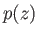and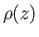be the pressure and density, respectively, as functions of altitude. Consider a packet of air that is in equilibrium with the surrounding air at some initial altitude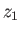, but subsequently moves to a higher altitude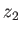. Thus, the packet's initial pressure and density are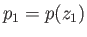and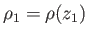, respectively. Now, at the higher altitude, the packet must adjust its volume in such a manner that its pressure matches that of the surrounding air, otherwise there would be a force imbalance across the packet boundary. It follows that the packet pressure at altitudeis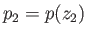. Assuming that the packet moves upward on a much faster time scale than that required for heat to diffuse across it (but still a sufficiently slow time scale that it remains in approximate pressure balance with the surrounding air), we would expect its internal pressure and density to be related according to the adiabatic gas law, (13.4). Thus, the packet's density at altitudeis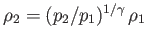. Now, if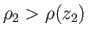then the packet is denser than the surrounding air. It follows that the packet's weight exceeds the buoyancy due to the atmosphere, causing the packet to sink back to its original altitude. On the other hand, if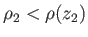then the packet is less dense than the surrounding air. It follows that the buoyancy force exceeds the packet's weight, causing it to rise to an even higher altitude. In other words, the atmosphere is unstable to vertical convection when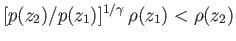for any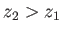: that is, when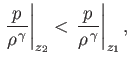(13.18)

for any. It follows that the atmosphere is only stable to vertical convection when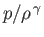is a monotonically increasing function of altitude. As is easily demonstrated, this stability criterion can also be written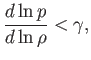(13.19)

or, making use of the ideal gas equation of state,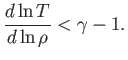(13.20)

Convection is triggered in regions of the atmosphere where the previous stability criterion is violated. However, such convection acts to relax these regions back to a marginally-stable state in whichis uniform: in other words, an adiabatic equilibrium.Next: Eddington Solar Model Up: Equilibrium of Compressible Fluids Previous: Adiabatic Atmosphere
Richard Fitzpatrick 2016-03-31